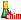$\definecolor{red}{RGB}{250,0,0}$ $\definecolor{black}{RGB}{0,0,0}$Search:

# The solubility of ionic substances

## Solution in water

A ionic substance is soluble in water if it dissociates to ions in water

Solution of sodium chloride: $Na^+Cl^-$ $\longrightarrow$ $Na^+$ $\color{red}+$ $Cl^-$ Solution of potassium sulfate: $(K^+)\color{red}_2$$SO_4^{2-} \longrightarrow$$\color{red}2$$K^+$$\color{red}+$$SO_4^{2-} Solution of aluminium chlorate: Al^{3+}(ClO_3^{-})$$\color{red}_3$ $\longrightarrow$ $Al^{3+}$ $\color{red}+$ $\color{red}3\color{black}ClO_3^{-}$

The formulas of undissolved substances display the number of ions as subscripts and behind their symbol I In the formulas of solutions, ions are separated by the symbol + and the number of ions is displayed before their symbol indicating that those ions are free!!

## Solubility rules

Following rules are useful :

In order of priority from top to bottom: 1) Ammonium $(NH_4^+)$, sodium $(Na^+)$ and potassium $(K^+)$ salts are soluble. 2) Nitrates $(NO_3^-)$, acetates $(C_2H_3O_2^-)$ and perchlorates $(ClO_4^-)$ are soluble. 3)Silver $(Ag^+)$, lead $(Pb^{2+})$ and mercury(I) $(Hg_2^{2+})$ salts are insoluble. 4) Chlorides $(Cl^-)$, bromides $(Br^-)$ and iodides $(I^-)$ are soluble. 5) Carbonates $(CO_3^{2-})$, sulfides $(S^{2-})$, oxides $(O^{2-})$ and hydroxides $(OH^-)$ are insoluble. 6) Sulfates $(SO_4^{2-})$ are soluble, except for calcium $(Ca^{2+})$ and barium $(Ba^{2+})$ sulfate.

### Examples:

Sodium carbonate is soluble, rule 1 > rule 5 $(Na^+)_2CO_3^{2-} \longrightarrow 2Na^+ + CO_3^{2-}$ Lead iodide is insoluble, because rule 3 > rule 4 $Pb^{2+}(I^-)_2)$Silver sulfate is insoluble, because rule 3 > rule 6 $(Ag^+)_2SO_4^{2-}$Nickel(II) sulfide is insoluble, because of rule 5 $Ni^{2+}S^{2-}$